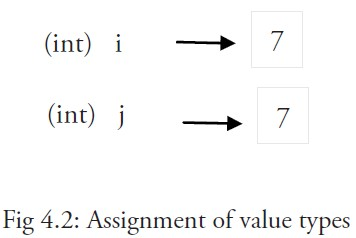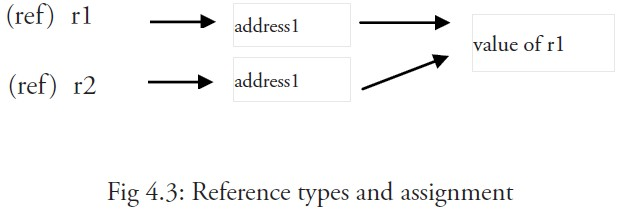# 4.4 变量

## 4.4.1 简介

``````var a, b *int
``````

``````var a int
var b bool
var str string
``````

``````var (
a int
b bool
str string
)
``````

``````a = 15
b = false
``````

``````a = b
``````

``````var identifier [type] = value
var a int = 15
var i = 5
var b bool = false
var str string = "Go says hello to the world!"
``````

``````var a = 15
var b = false
var str = "Go says hello to the world!"
``````

``````var (
a = 15
b = false
str = "Go says hello to the world!"
numShips = 50
city string
)
``````

``````var n int64 = 2
``````

``````var (
HOME = os.Getenv("HOME")
USER = os.Getenv("USER")
GOROOT = os.Getenv("GOROOT")
)
``````

``````a := 1
``````

``````package main

import (
"fmt"
"os"
)

func main() {
var goos string = os.Getenv("GOOS")
fmt.Printf("The operating system is: %s\n", goos)
path := os.Getenv("PATH")
fmt.Printf("Path is %s\n", path)
}
``````

## 4.4.2 值类型和引用类型## 4.4.3 打印

``````func Printf(format string, list of variables to be printed)
``````

``````fmt.Print("Hello:", 23)
``````

## 4.4.4 简短形式，使用 := 赋值操作符

a 和 b 的类型（int 和 bool）将由编译器自动推断。

``````func main() {
var a string = "abc"
fmt.Println("hello, world")
}
``````

``````var a, b, c int
``````

(这是将类型写在标识符后面的一个重要原因)

``````a, b, c = 5, 7, "abc"
``````

``````a, b, c := 5, 7, "abc"
``````

(在 Go 语言中，这样省去了使用交换函数的必要)

`_` 实际上是一个只写变量，你不能得到它的值。这样做是因为 Go 语言中你必须使用所有被声明的变量，但有时你并不需要使用从一个函数得到的所有返回值。

## 4.4.5 init 函数

``````package trans

import "math"

var Pi float64

func init() {
Pi = 4 * math.Atan(1) // init() function computes Pi
}
``````

``````package main

import (
"fmt"
"./trans"
)

var twoPi = 2 * trans.Pi

func main() {
fmt.Printf("2*Pi = %g\n", twoPi) // 2*Pi = 6.283185307179586
}
``````

init 函数也经常被用在当一个程序开始之前调用后台执行的 goroutine，如下面这个例子当中的 `backend()`

``````func init() {
// setup preparations
go backend()
}
``````

``````package main

var a = "G"

func main() {
n()
m()
n()
}

func n() { print(a) }

func m() {
a := "O"
print(a)
}
``````

``````package main

var a = "G"

func main() {
n()
m()
n()
}

func n() {
print(a)
}

func m() {
a = "O"
print(a)
}
``````
``````package main

var a string

func main() {
a = "G"
print(a)
f1()
}

func f1() {
a := "O"
print(a)
f2()
}

func f2() {
print(a)
}
``````